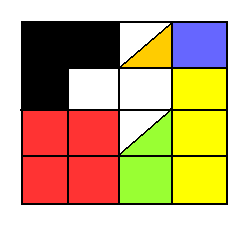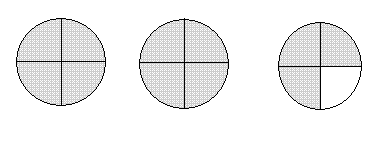# Fractions and Mixed Numbers Grade 6 Math Questions and Problems With Solutions and Explanations

Detailed solutions and full explanations to grade 6 fractions and mixed numbers questions are presented.

 Note: In this page, fractions are written with diagonal bars. For example 1/3 is the fraction whose numerator is 1 and denominator is 3. 6 1/3 is a mixed number meaning 6 + 1/3. What fraction of the large square is red? What fraction of the large square is blue? What fraction of the large square is orange? What fraction of the large square is green? What fraction of the large square is black? What fraction of the large square is yellow?Solution The large square is made up of 16 small squares. There are 4 red small squares. Hence the fraction of the large square that is red is given by 4 / 16 Divide numerator and denominator by 4 to reduce the fraction to = 1 / 4 There is one blue square. Hence the fraction of the large square that is blue is given by 1 / 16 There is one half of a square that is orange. Hence the fraction of the large square that is orange is given by half of 1 / 16 = (1/2) (1/16) = 1 / 32 There is one and one half of a small square that are green. Hence the fraction of the large square that is green is given by 1 / 16 + (1/2)(1 / 16) = 1 / 16 + 1 / 32 = 2 / 32 + 1 / 32 = 3 / 32 , common denominator and addition There are 3 small square that are black. Hence the fraction of the large square that is black is given by 3 / 16 There are 3 small square that are yellow. Hence the fraction of the large square that is yellow is given by 3 / 16 What fraction is the shaded part?Solution There are two whole items and 3 / 4 of one item. Hence the part that is shaded is given by 2 + 3/4 = 2 3/4 (mixed number) Which point on the number line represents 1 1/5?Solution Note that 1 1/5 is larger than 1 and smaller than 2. Each small division on the number line represent 1 / 10. Hence point R represents 1 and 2/10 = 1 2/10 = 1 1/5 , reduce fraction 2/10 = 1/5 Point R represents 1 1/5 3 1/2 + 5 1/2 = Solution Add whole numbers together and fractions together 3 1/2 + 5 1/2 = (3 + 5) + (1/2 + 1/2) = 8 + 2/2 = 8 + 1 = 9 1/2 + 1/14 = Write to the same denominator before you add fractions 1/2 + 1/14 = 7 / 14 + 1 / 14 , common denominator 14 = 8 / 14 , add numerators = 4 / 7 , divide numerator and denominator by 2 to reduce fraction 1/3 - 1/12 = Solution Rewrite to the same denominator before you subtract fractions 1 / 3 - 1 / 12 = 4 / 12 - 1 / 12 , common denominator = 3 / 12 , subtract numerator = 1 / 4 , divide numerator and denominator to reduce fraction one half is the same as? Solution one half is written as 1 / 2 two quarters is written as 2 / 4 = 1 / 2 , divide numerator and denominator to reduce fraction Hence one half is the same as two quarters. Which two fractions are not equivalent? 1/2 and 2/4 4/3 and 8/6 1/5 and 3/15 2/3 and 8/9 Solution 2 / 4 is equivalent to 1 / 2 since 2 / 4 = 1 / 2 after division of numerator and denominator of 2 / 4 by 2 8 / 6 is equivalent to 4 / 3 since 4 / 3 = 8 / 6 after multiplication of numerator and denominator of 4 / 3 by 2 1 / 5 is equivalent to 3 / 15 since 1 / 5 = 3 / 15 after multiplication of numerator and denominator of 1 / 5 by 3 2 / 3 and 8 / 9 are not equivalent. 5 2/3 + 5 1/2 = Solution Add whole numbers together and fractions together 5 2/3 + 5 1/2 = (5 + 5) + (2 / 3 + 1 / 2) = 10 + (4 / 6 + 3 / 6) , common denominator for fractions = 10 + 7/6 = 10 + 6/6 + 1/6 , write 7/6 as 6/ + 1/6 = 10 + 1 + 1/6 = 11 1/6 , simplify Order from least to greatest: 8/9 , 17/18 , 2/3 , 7/6 . Solution It is easier to campare fractions with the same denominator and so we first write all given fractions to the same denominator 18 8 / 9 = 16 / 18 17 / 18 = 17 / 18 2 / 3 = 12 / 18 7 / 6 = 21 / 18 We now order the fractions from least to greatest 2 / 3 , 8 / 9 , 17 / 18 , 7 / 6 Which fraction is closest to 1? . 10/11 11/10 9/11 -9/10 Solution We first write all given fractions to the same denominator 110 and see which is closer to 110 / 110 which is equal to 1. 10 / 11 = 100 / 110 11 / 10 = 121 / 110 9 / 11 = 90 / 110 - 9 / 10 = - 99 / 110 Now that they have the same denominator, the closest to 1 is the one whose numerator is closer to 110. Hence 10 / 11 is the closest to 1. 5/2 � 2/5 = . Solution 5 / 2 � 2 / 5 = 5 / 2 � 5 / 2 = 25 / 4 = 24 / 4 + 1 / 4 = 6 1/4 5 � 1/5 = Solution 5 � 1 / 5 = 5 � 5 / 1 = 25 2/5 � 7/8 = Solution 2 / 5 � 7 / 8 = (2 � 7) / (5 � 8) = (1 � 7) / (5 � 4) , simplify = 7 / 20 Write the mixed number 7 7/8 as a fraction. Solution 7 7/8 = 7 � 8 / 8 + 7 / 8 = (7 � 8 + 7) / 8 = 63 / 8 Write the fraction 31/8 as a mixed number. Solution 31 / 8 = (24 + 7) / 8 = 3 7/8 3 � 1/4 = Solution 3 � 1 / 4 = 3 / 1 � 1 / 4 = (3 � 1) / (4 � 1) = 3 / 4 3 1/4 � 5 1/3 = Solution Change the mixed numbers 3 1/4 and 5 1/3 to fractions and then divide. 3 1/4 = 13 / 4 5 1/3 = 16 / 3 hence 3 1/4 � 5 1/3 = 13 / 4 � 16 / 3 = 13 / 4 � 3 / 16 = 39 / 64 4 2/7 � 5 3/5 = Solution Change the mixed numbers 4 2/7 and 5 3/5 to fractions and then multiply. 4 2/7 = 30 / 7 5 3/5 = 28 / 5 hence 4 2/7 � 5 3/5 = 30 / 7 � 28 / 5 = (30 � 28) / (7 � 5) = 24 To have F + 2 5/7 = 4, F must be equal to Solution In order to have F + 2 5/7 = 4, F must be equal to F = 4 - 2 5/7 = 2 - 5/7 = 14 / 7 - 5 / 7 = 9 / 7 = 1 2/7 Tom runs 3/4 of an hour every Monday, 30 minutes every Tuesday, half an hour every Wednesday, 1 1/4 hours every Thursday and 2/3 of an hour on Friday. How many hours does Tom run from Monday to Friday? . Solution Find the time in minutes for each day. Monday: 3 / 4 of an hour = (3 / 4) � 60 = 180 / 4 = 45 minutes Tuesday: 30 minutes Wednesday: half an hour = (1 / 2) � 60 = 30 minutes Thursday: 1 1/4 hours = 1 hour + (1 / 4) of an hour = 60 + 60 / 4 = 75 minutes Friday: 2 / 3 of an hour = (2 / 3) � 60 = 40 minutes Add the times for all 5 days. 45 + 30 + 30 + 75 + 40 = 220 minutes = (180 + 40) minutes = 3 hours and 40 minutes Order from least to greatest: 5 3/4 , 3 4/5 , 3 1/5 , 4 5/6. Solution The mixed number with the smallest whole number is the smallest. Hence 3 1/5 is the smallest and 3 4/5 is the second smallest. The next largest is 4 5/6 and the largest is 5 3/4. Hence the order from least to greatest is given by 3 1/5 , 3 4/5 , 4 5/6 , 5 3/4 Order from least to greatest: 7 2/3 , 7 3/5 , 7 3/4 , 7 6/11. Solution The given mixed numbers all have the same whole number and the fractional parts are not easy to compare either. The best way to compare these mixed numbers is to compare their fractional parts and to compare their fractional parts we need to write them to the lowest common denominator 660. 2 / 3 = 440 / 660 3 / 5 = 369 / 660 3 / 4 = 495 / 660 6 / 11 = 360 / 660 It is easier to compare fractions with common denominator. Hence the order from least to greatest of the given mixed numbers is 7 6/11 , 7 3/5 , 7 2/3 , 7 3/4 What fraction of 1 hour is 50 minutes? Solution A fraction is a part of a whole. 50 minutes = (50 / 60) � 60 = 50 / 60 of one hour = 5 / 6 of one hour. Hence 50 minutes is 5 / 6 of an hour. 1/3 is 1/8 of what number? Solution Let the number be n. Rewrite the given question in mathematical terms 1 / 3 = 1 / 8 of n 1 / 3 = (1 / 8) � n 1 / 3 = n / 8 The above may be written with fractions having common denominator. (1 � 8) / (3 � 8) = (n � 3) / (8 � 3) 8 / 24 = 3 n / 24 The above fractions have common denominator and they are equal if the numerators are equal. Hence 8 = 3 (8 / 3) = 3 n , hence n = 8 / 3

More Middle School Math (Grades 6, 7, 8, 9) - Free Questions and Problems With Answers
More High School Math (Grades 10, 11 and 12) - Free Questions and Problems With Answers
More Primary Math (Grades 4 and 5) with Free Questions and Problems With Answers